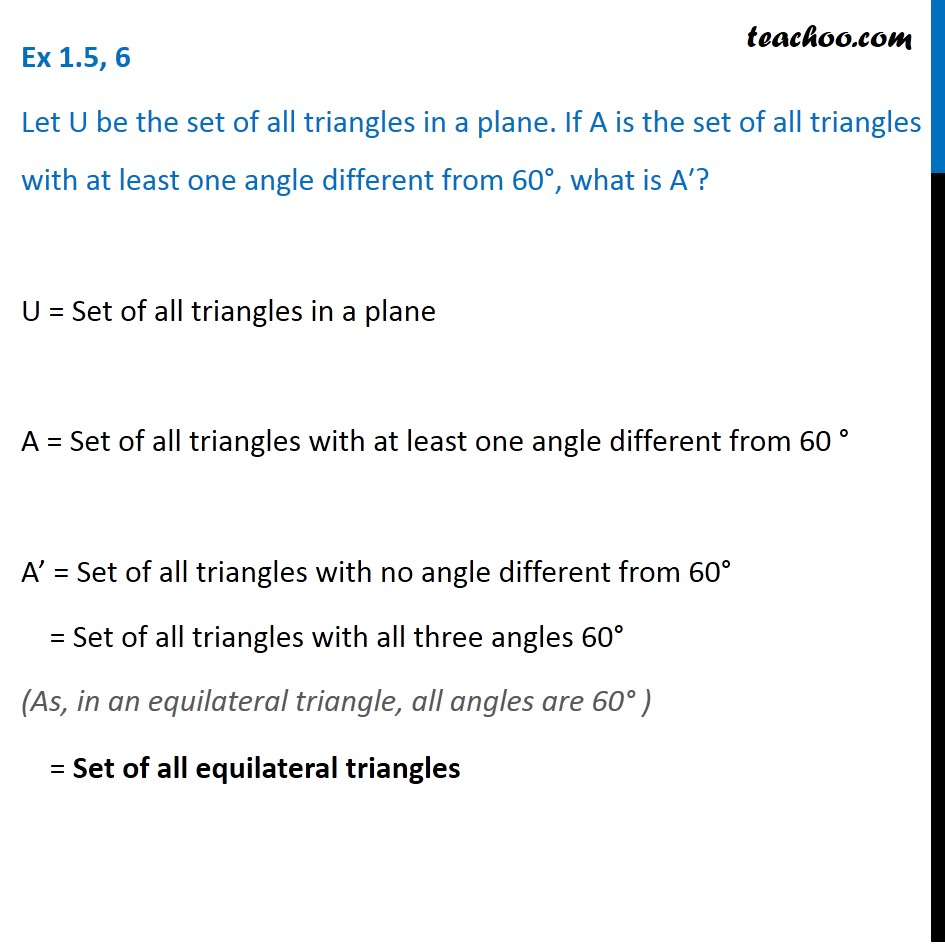1. Chapter 1 Class 11 Sets
2. Concept wise
3. Complement of set

Transcript

Ex 1.5, 6 Let U be the set of all triangles in a plane. If A is the set of all triangles with at least one angle different from 60°, what is A′? U = Set of all triangles in a plane A = Set of all triangles with at least one angle different from 60 ° A’ = Set of all triangles with no angle different from 60° = Set of all triangles with all three angles 60° = Set of all equilateral triangles

Complement of set